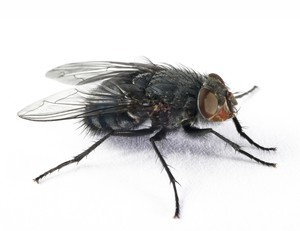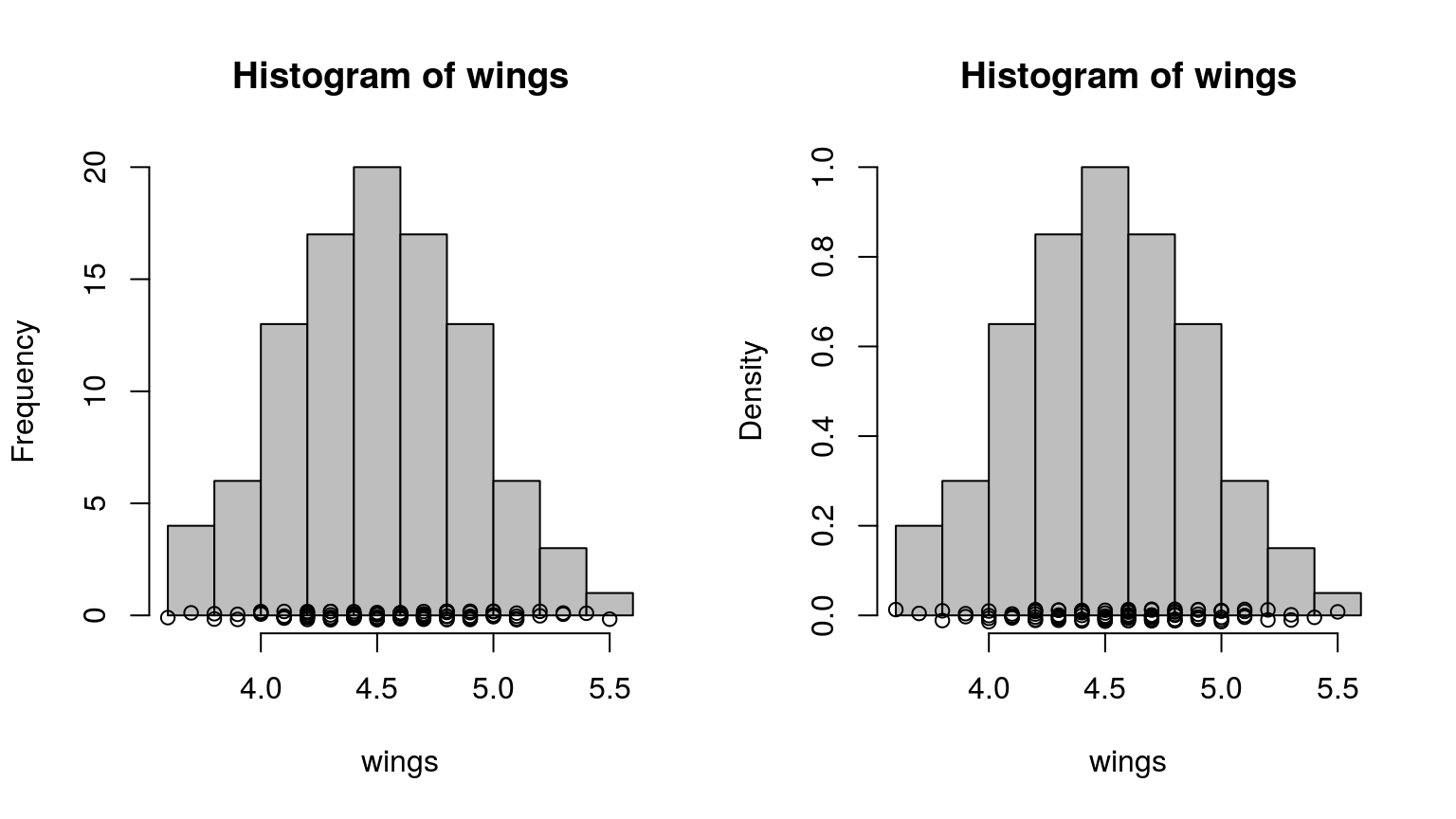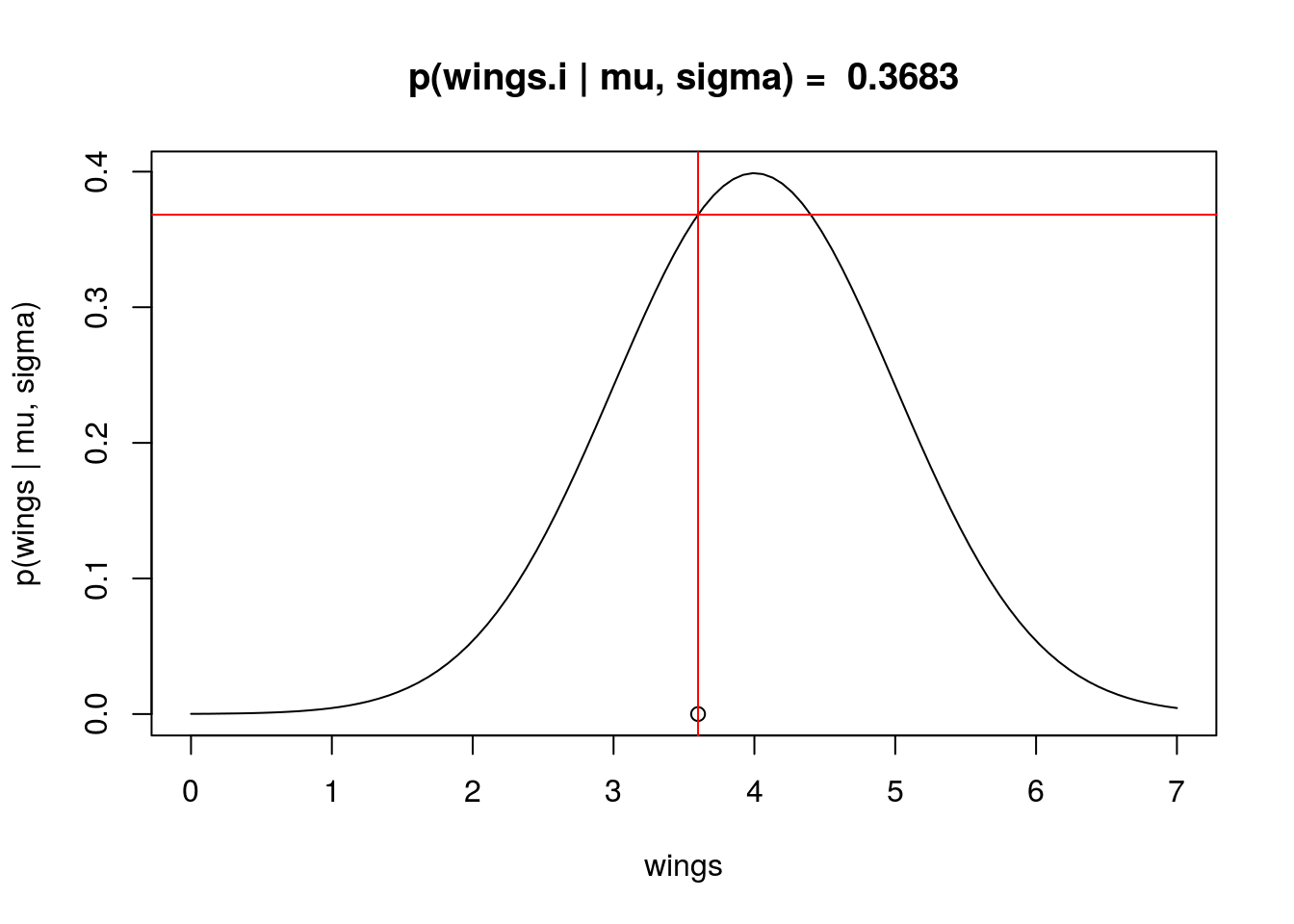The purpose of this lesson is to explain the concept of probability density and likelihood, using a simple example of Normal probability density function.

# 1 The data

We will use data on housefly wing length [mm], which you can find here.

The original data are from Sokal & Hunter (1955) A morphometric analysis of DDT-resistant and non-resistant housefly strains Ann. Entomol. Soc. Amer. 48: 499-507.To fetch the data directly from the web, run:

wings <- read.table("http://goo.gl/4lPBG6", header=FALSE)[,1]*0.1

Let’s examine the data:

wings
##    3.6 3.7 3.8 3.8 3.9 3.9 4.0 4.0 4.0 4.0 4.1 4.1 4.1 4.1 4.1 4.1 4.2
##   4.2 4.2 4.2 4.2 4.2 4.2 4.3 4.3 4.3 4.3 4.3 4.3 4.3 4.3 4.4 4.4 4.4
##   4.4 4.4 4.4 4.4 4.4 4.4 4.5 4.5 4.5 4.5 4.5 4.5 4.5 4.5 4.5 4.5 4.6
##   4.6 4.6 4.6 4.6 4.6 4.6 4.6 4.6 4.6 4.7 4.7 4.7 4.7 4.7 4.7 4.7 4.7
##   4.7 4.8 4.8 4.8 4.8 4.8 4.8 4.8 4.8 4.9 4.9 4.9 4.9 4.9 4.9 4.9 5.0
##   5.0 5.0 5.0 5.0 5.0 5.1 5.1 5.1 5.1 5.2 5.2 5.3 5.3 5.4 5.5
par(mfrow=c(1,2))
hist(wings, freq=TRUE, col="grey")
points(wings, jitter(rep(0, times=100), factor=10))
hist(wings, freq=FALSE, col="grey")
points(wings, jitter(rep(0, times=100), factor=0.7))# 2 The Normal model

This is our formal model definition:

$p(x) = \frac{1}{\sqrt{2\pi}\sigma}e^{-(x-\mu)^2/(2\sigma^2)}$

where $$p()$$ is probability density function (also known as PDF). The function has two parameters: $$\mu$$ (mean) and $$\sigma$$ (standard deviation).

For $$\mu = 0$$ and $$\sigma = 1$$ the model looks like this:

  curve(dnorm, from=-4, to=4, ylab="p(x)")Remember:

• Probability density is different from probability.

• Probability density is denoted by $$p$$, while probability is $$P$$.

• Probability density can be higher than 1.

• Probability density function must integrate to 1.

• Probability distribution is a very ambiguous and confusing term.

# 3 Fitting the model to the wing data

KEY PROBLEM: How do we decide which parametrization is the best for our data?## 3.1 Likelihood - single data point

The likelihood function is the density evaluated at the data $$x_1$$, … ,$$x_n$$, viewed as a function of model parameters ($$\mu$$ and $$\sigma$$ in case of the Normal model). We write it as $$L(\mu, \sigma | x) = p(x | \mu, \sigma)$$.

Calculation of likelihood in R is easy! The R functions dnorm(), dpois(), dunif(), dbinom(), dgamma(), dbeta() etc. are exactly for that!

Example: What is the likelihood of the first data value in the wings dataset, given the $$Normal(\mu=4, \sigma=1)$$ model?

my.mean = 4
my.sd = 1

Here is the data point that we will examine. Note that I use the letter i to denote index:

wings.i <- wings
wings.i
##  3.6

Here is how you calculate the likelihood for the data point wings.i using the function dnorm:

L <- dnorm(x=wings.i, mean=my.mean, sd=my.sd)
L
##  0.3683

Let’s plot it:

  curve(dnorm(x, my.mean, my.sd), from=0, to=7,
ylab="p(wings | mu, sigma)", xlab="wings",
main=paste("p(wings.i | mu, sigma) = ", round(L, 4)))
points(wings.i, 0)
abline(v=wings.i, col="red")
abline(h=L, col="red")# 4 Likelihood - whole dataset

Basic probability theory tells us that:

$P(A \cap B) = P(A) \times P(B|A) = P(B) \times P(A|B)$

Where $$P(A \cap B)$$ is joint probability, associated with AND, meaning “at the same time”.

The problem is that joint probability for more than two events, e.g. $$P( A \cap B \cap C \cap D )$$, can be almost impossible to calculate, with the exception of A and B being independent! Then: $P(A \cap B) = P(A) \times P(B)$ and hence $P( A \cap B \cap C \cap D ) = P(A) \times P(B) \times P(C) \times P(D)$

It follows that it is useful to subject probability density $$p()$$ to the same rules as probability $$P()$$. Hence, we can calculate the likelihood of the whole dataset as a product of likelihoods of all individual data points!

  wings
##    3.6 3.7 3.8 3.8 3.9 3.9 4.0 4.0 4.0 4.0 4.1 4.1 4.1 4.1 4.1 4.1 4.2
##   4.2 4.2 4.2 4.2 4.2 4.2 4.3 4.3 4.3 4.3 4.3 4.3 4.3 4.3 4.4 4.4 4.4
##   4.4 4.4 4.4 4.4 4.4 4.4 4.5 4.5 4.5 4.5 4.5 4.5 4.5 4.5 4.5 4.5 4.6
##   4.6 4.6 4.6 4.6 4.6 4.6 4.6 4.6 4.6 4.7 4.7 4.7 4.7 4.7 4.7 4.7 4.7
##   4.7 4.8 4.8 4.8 4.8 4.8 4.8 4.8 4.8 4.9 4.9 4.9 4.9 4.9 4.9 4.9 5.0
##   5.0 5.0 5.0 5.0 5.0 5.1 5.1 5.1 5.1 5.2 5.2 5.3 5.3 5.4 5.5
  L <- dnorm(x=wings, mean=my.mean, sd=my.sd)
L
##    0.3683 0.3814 0.3910 0.3910 0.3970 0.3970 0.3989 0.3989 0.3989 0.3989
##   0.3970 0.3970 0.3970 0.3970 0.3970 0.3970 0.3910 0.3910 0.3910 0.3910
##   0.3910 0.3910 0.3910 0.3814 0.3814 0.3814 0.3814 0.3814 0.3814 0.3814
##   0.3814 0.3683 0.3683 0.3683 0.3683 0.3683 0.3683 0.3683 0.3683 0.3683
##   0.3521 0.3521 0.3521 0.3521 0.3521 0.3521 0.3521 0.3521 0.3521 0.3521
##   0.3332 0.3332 0.3332 0.3332 0.3332 0.3332 0.3332 0.3332 0.3332 0.3332
##   0.3123 0.3123 0.3123 0.3123 0.3123 0.3123 0.3123 0.3123 0.3123 0.2897
##   0.2897 0.2897 0.2897 0.2897 0.2897 0.2897 0.2897 0.2661 0.2661 0.2661
##   0.2661 0.2661 0.2661 0.2661 0.2420 0.2420 0.2420 0.2420 0.2420 0.2420
##   0.2179 0.2179 0.2179 0.2179 0.1942 0.1942 0.1714 0.1714 0.1497 0.1295
  prod(L)
##  1.658e-50

This is a ridiculously small number! Which is why we have the Negative Log Likelihood, also known as the deviance:

  - sum(log(L))
##  114.6

We can encapsulate it into a single function:

  deviance.function <- function(x, mean, sd)
{
LL <- dnorm(x=x, mean=mean, sd=sd, log=TRUE) # note the log!!!
deviance <- - sum(LL)
return(deviance)
}

# it's a function of model parameters, so try to play
# around with different paramter values
deviance.function(wings, mean=0, sd=1)
##  1135

Deviance (negative log-likelihood) can be then minimized (likelihood is maximized) in order to find the most likely model parameters - these are the Maximum Likelihood Estimators (MLE) of model parameters.

One can use optim() for automatic likelihood optimization.

# 5 Exercise

Find and plot the MLE of the Normal model and the ‘wings’ data. Use the following functions and scripts:

 deviance.function()
hist()
curve()

# 6 For further exploration

There are other functions derived from probability density functions. I have a tutorial post on that.

Type ?pnorm, ?qnorm or ?rnorm and check it out: